## 3.7.2 More Realistic Travel-Time Model

In most practical situations, the effective travel speed of urban response units depends on travel distance: longer trips, in general, are taken at a higher average speed than are shorter trips. It is therefore desirable to develop expressions for E[T] that take into consideration some types of functional relationships between travel time and travel distance [unlike expressions (3.81) and (3.86), which assumed that effective travel speed remains constant with distance]. One plausible model is the following. Let us assume that urban service vehicles responding to a call, first go through an acceleration stage (perhaps while maneuvering their way through side streets, turns, etc.) until they reach a cruising speed that they maintain through the middle stage of the trip (while, perhaps, traveling on highways, thoroughfares, etc.) up to the final stage of it, during which they decelerate to a stop. Let us further assume that during the initial and final stages, vehicles accelerate (or decelerate) at a constant rate of a miles/min2 and that during the middle stage, travel is at a constant cruising speed of v, miles/min.
For trips of length less than 2dc, (where dc, = v2c/2a is the distance needed to reach cruising speed) the cruising speed will neverbe reached; this is not the case when the travel distance D is greater than 2dc. Using the well-known physical relationships for accelerated and constant speed travel (D = at2c/2 and D = vt), it is then easy to conclude that the conditional expected travel time E[T | D = d] for any given travel distance is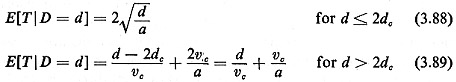One can obviously think of many other physical scenarios that would lead to different expressions for E[T | D = d]. A considerable amount of field data, however, suggests that (3.88) and (3.89) often provide truly excellent approximations for many urban services-see, for instance, [KOLE 75, JARV 75, HAUS 75].
An expression for the unconditional expected travel time, E[T], can now be written: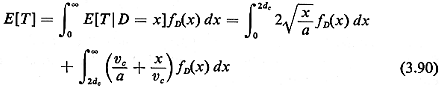In order to evaluate the two integrals in (3.90) it is necessary to know the pdf for the travel distance, fd(x).

Example 13: Expected Travel Time in a Square District

Consider a 1- by 1-mile-square fire district with a firehouse located at its center. Fire alarms are distributed independently and uniformly within the district and the travel metric is right-angle with directions of travel parallel to the sides of the square. Measurements have shown that the "cruising speed" for fire engines is vx = vy = vc = 30 miles/hr and vehicle acceleration and deceleration (as described above) is a = 0.5 miles/min2; that is, it takes, on the average, about I minute of travel for the fire engines to accelerate up to (or decelerate down from) cruising speed. (These values are rather typical, as field data show-see below.) It follows that dc = V2c/2a = 0.25 mile.

From (3.88) and (3.89) we then have (in minutes)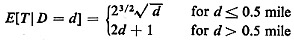From the fact that Dx and Dy the distances traveled in the east-west and north-south directions, respectively, are independent random variables distributed uniformly between 0 and 1/2 mile, it is easy to show, using the techniques of this chapter (see Problem 3.9), that for D = Dx + Dy we havefor the average travel time in responding to a fire alarm in this district.

Unfortunately, the pdf for the travel disance fD(x) is often difficult to obtain, either theoreticallly or from field data. The following approximate expression for E[T|D=d] is then often used in order to overcome this problem: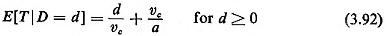This expression is compared with (3.88) and (3.89) in Figure 3.30. Note that (3.92) is a "conservative" model for
E[T | D = d] in the sense that it provides an upper bound for (3.88) and (3.89) and is also a good approximation to it for all values of D, when 2dc is relatively small by comparison to the distances that a response unit usually travels. The physical interpretation of (3.92) is also simple: a fixed amount of time (= vc/a) is spent getting ready for each trip and then the trip takes place at a constant travel speed,9 vc. Obviously, the advantage of (3.92) is that fD(x) is no longer necessary to develop a simple expression for the unconditional travel time E[Y]. For we now have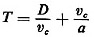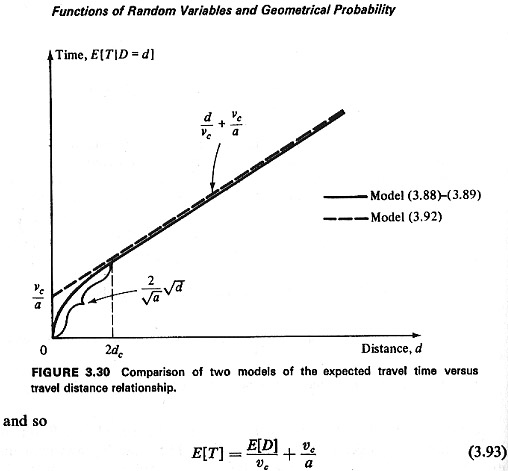Example 13: (continued)

For our I- by 1-mile-square-district example, it is obvious by inspection that E[D] = 0.5 mile (cf. Table 3-1). It follows that, with v = 0.5 mile/min2 and a = 0.5 mile/min2, E[T] = 2 minutes, or only about 2 seconds more than our earlier exact estimate! The very close agreement between the two estimates may seem surprising in view of the fact that 2dc = 0.5 mile in this case, or 50 percent of the full range of values of the travel distance D.

The example above is not atypical. Estimates of E[T] obtained through (3.92) and (3.93) are usually very close to estimates obtained through the time-consuming approach summarized by (3.88)-(3.90) for the values of v, and a one encounters in urban service applications.
Table 3-2 lists four sets of measured values of v, and a for four different cities in the United States. The data were collected by the fire departments in these cities [HAUS 75]. Note the similarity of the values for the cruising speeds. From Table 3-2 it can also be inferred that the constant v,la typically adds about 0.5 to 1.0 minute to E[T] in (3.93).
In this section we have thus concluded that: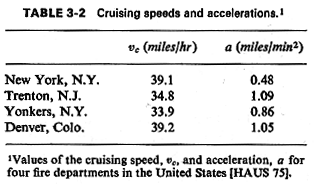The travel time/travel distance relationship (3.92) greatly simplifies the calculation of E[T] and provides excellent approximations to results produced through more complicated analyses.

It should be noted that our earlier results regarding variation of E[T] with district geometries apply unaltered in the context of (3.93). This is because of the continued linear relationship between D and T, which is augmented in (3.93) only by an additive constant, a term that does not affect variational analyses of district geometries. Even when cruising travel speed depends on direction of travel, i.e., when vx.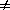vy., the addition of a constant to the travel time will not affect the variations of travel times with district designs. Most important, optimal designs remain unchanged.

9 It is also possible to have this effective travel speed depend on the direction of travel (e.g., as in the case of right-angle travel).